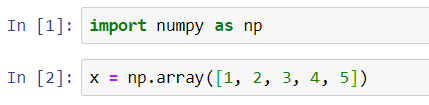NumPy provides us with all standard arithmetic operations such as addition, subtraction, multiplication, and division, etc. which we can perform in an array.

Let us begin by working with those functions.This function will add n to every element of array x.#### np.subtract(x, n)

This function will subtract n from each and every element of array x.#### np.negative(x)

This function will change the polarity of every element in array x.#### np.divide(x, n)

This function will divide each and every element of array x by n.#### np.floor_divide(x, n)

This function will perform floor division of each and every element of array x by n.#### np.power(x, n)

This function will calculate the power on n (exponentiation) for each and every element of array x.#### np.mod(x)

This function will calculate the remainder value when each and every element of array x is divided by n.